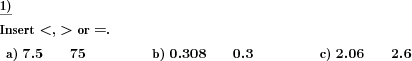Custom math worksheets at your fingertips# Details for problem "Insert relational operator"

Quickname: 7760

Elementary School, Primary School, Junior High School, Middle School, High School.

## Summary

Insert the correct relational symbol between two decimals.

## Example## Description

A series of pairs of decimal fractions is presented. The task is to determine whether these numbers are equal or not, if not, which one is greater, and insert the correct relational operator "less than", "greater than", or "equal" in the blank space between the numbers.

The way the pairs of numbers, a and b, are chosen can be selected from different algorithms:

• n and m are natural numbers from 1 to 9, a is n+m/10, b is n+m/100, i.e. m will occur in the tenth position of the first number a and in the hundredth position of the second number b. Example: 4.5 and 4.05.
• a is a natural number in the range from 11 to 99, b is a/10, e.g. 45 and 4.5.
• a and b are numbers with three decimals, a is by 0.001 larger than b.
• a is a number with one decimal place, b is b+0.001,..,0.09, e.g. 4.1 and 4.103.
• a and b have the same digits, but the order is different, e.g. 4.21 and 40.12.
• a and b are equal, but b is shown with a trailing zero, e.g. 4.2 and 4.20.

For each type of problem, the number of occurrences can be chosen. The problems of different types may occur grouped or shuffled, this is selectable.

Numbers may be positive or negative, this is configurable.

Download free printable worksheets for this math problem here. The worksheet contains the problems only, the solution sheet includes the answers. Just click on the respective link.

•Worksheet 1Solution sheet with answers
•Worksheet 2Solution sheet with answers
•Worksheet 3Solution sheet with answers

If you can not see the solution sheets for download, they may be filtered out by an ad blocker that you may have installed. If this is the case, please allow ads for this page and reload the page. The solution sheets will then reappear.

• Do these sample worksheets do not really fit?
• Do you need more math worksheets, with a different level of difficulty?
• Would you like to combine different problems on a worksheet and adjust them to your needs?
• As a teacher, you can put together your own worksheets using the automatically generated math problems provided.
With a free initial credit, you can start creating your own math worksheets in a few minutes.

You can try it for free! Register here, to create custom worksheets now!

## Customization options for this problem

Parameter
Possible values
# n,m natural,a=n+m/10,b=n+m/100
0, 1, 2, 3, 4, 5, 6
# a=11-99, b=a/10
0, 1, 2, 3, 4, 5, 6
# a,b three decimals, a greater by 0.001
0, 1, 2, 3, 4, 5, 6
# a one decimal, b three, tenths=0
0, 1, 2, 3, 4, 5, 6
# a und b jumbled digits
0, 1, 2, 3, 4, 5, 6
# a,b same, b with trailing zero
0, 1, 2, 3, 4, 5, 6
Shuffle
Yes, No
Positive/Negative
positive, across origin, positive/negative, negative

## Similar problems

Remark
Description
Similar problem with fractions
Insert the correct relational symbol between two fractions
Simpler variant, with natural numbers
Insert the correct relational symbol between two numbers.Deutsche Version dieser Aufgabe
These informational pages with samples describe math problems that can be combined on custom math worksheets with solutions for home and K-12 school use.
Deutsche Seiten
×